# 什么是迁移学习

Transfer Learning Setting（迁移学习设定） 相关领域 源空间标签是否存在 目标空间标签是否存在 任务
Inductive transfer learning(归纳迁移学习) 多任务学习 存在 存在 回归或者分类
Inductive transfer learning(归纳迁移学习) 自我启发式学习 不存在 存在 回归或者分类
Transductive transfer learning(归纳迁移学习) 空间自适应，采样偏差，边缘分布变换 存在 不存在 回归或者分类
Unsupervised transfer learning(无监督迁移学习) 不存在 不存在 聚类或者降维

# 领域自适应问题

• 数据分布的角度：源域和目标域的概率分布相似（最小化概率分布距离）
• 特征选择的角度：源域和目标域共享某些特征（选择出公共特征）
• 特征变化的角度：源域和目标域共享某些子空间（把两个域变换到相同的子空间）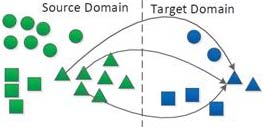## 概率分布适配

• 假设: $P(X_S) \neq P(X_t)$
• 假设: $P(y_S | X_S) \neq P(y_t | X_t)$
• 假设: $P(X_S,y_S) \neq P(X_t,y_t)$### 边缘分布适配

• 迁移成分分析（Transfer Component Analysis），其优化目标为$$min Dist(\varphi (X_S),\varphi(X_T)) + \lambda \Omega(\lambda)$$

$$s.t. constraints~on~\varphi(X_S) and \varphi(X_T)$$

• 最大均值差异（Maximum Mean Discrepancy） $$Dist(P(X_S),P(X_T)) = ||\frac{1}{n_S} \sum_{i=1}^{n_S} \Phi (x_{S_{i}}) - \frac{1}{n_T} \sum_{j=1}^{n_T} \Phi(x_{S_{j}})||_{H}$$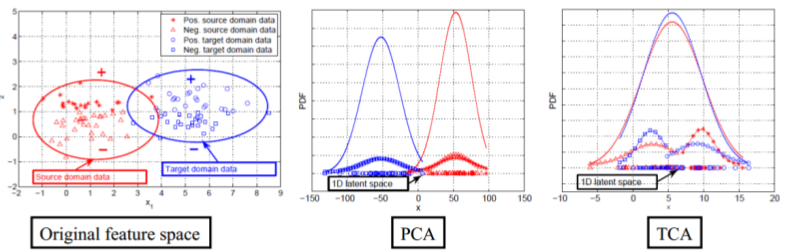• 迁移成分分析（TCA）的扩展
• Adaptating Component Analysis（ACA）$max~\dfrac{tr(HK_{X}HL_{\Phi})}{tr(HK_{M}HL_{\Phi})}$ 也就是最小化MMD，同时维持迁移过程之中目标域的结构
• Domain Transfer Multiple Kernel Learning 多核MMD
• Deep Domain Confusion 把MMD加入到神经网络中
• Distribution-Matching Embledding（DME）先计算变换矩阵，在进行映射
• Central Moment Discrepancy（CMD）k阶MMD

### 条件分布适配

Domain Adaptation of Conditional Probability Models via Feature Subsetting

$$such~that~dist(D,D’|S,D’) \leq \epsilon$$

Conditional Transferrable Components (CTC)### 联合分布适配

• Joint Geometrical and Statistical Alignment（JGSA）加入类间距、类内距和标签适配
• 或者加入结构不变性控制
• 目标域选择

• 当$\mu \rightarrow 0$，表示边缘分布更占优，应该优先适配
• 当$\mu \rightarrow 1$，表示条件分布更占优，应该优先适配

$$s.t.~ A^TXHX^TA = I, ~0\leq \mu \leq 1$$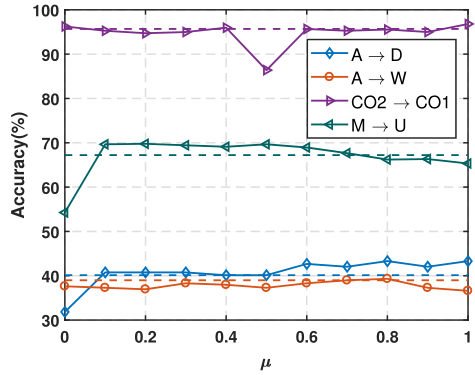• 求解源域和目标域整体的A距离
• 对目标域聚类，计算源域和目标域每个类的A距离
• 计算上述两个距离的比值，也就是平衡因子

### 总结

• 数据整体差异性大 (相似度较低),边缘分布更重要
• 数据整体差异性小 (协方差漂移),条件分布更重要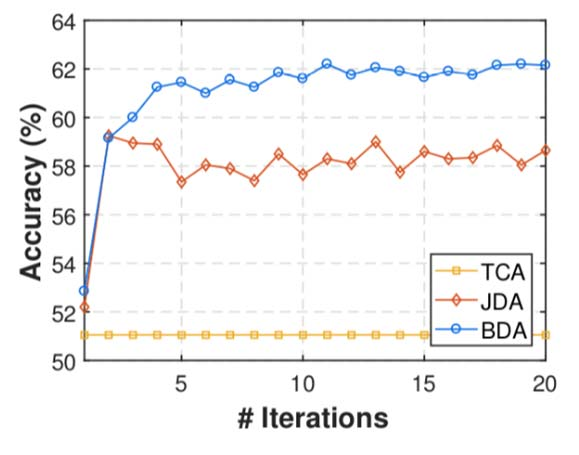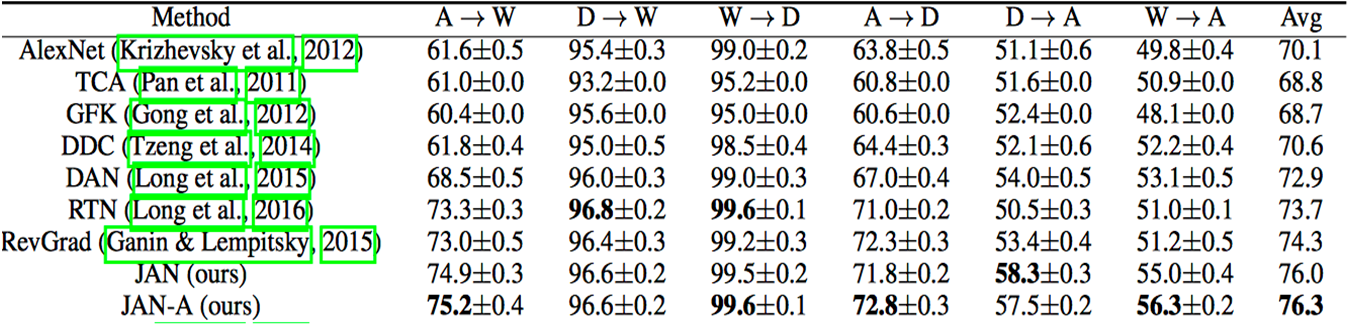## 特征选择法• Joint feature selection and subspace learning 特征选择/变换+子空间学习
• 优化目标:$$min_A ||A||_{2,1} + \mu tr(A^TXLX^TA), s.t. A^TXDX^TA = I$$
• Transfer Joint Matching (TJM) MMD分布适配+源域样本选择
• 优化目标: $$\min_{A^TKHK^TA = I} tr(A^TKHK^TA)+\mu (||A_s||_{2,1}+||A_t||_F^2)$$
• Feature Selection and Structure Preservation (FSSL) 特征选择+信息不变性
• 优化目标:$$\min_{P,Z,E} ||P||_{2,1} + \frac{\mu}{2} tr(P^TXLX^TP) + \frac{\beta}{2} ||Z||^2_F + \gamma ||E||_1 ,~~ s.t. P^TX_sZ+E, P^TXDX^TP = I$$

• 从源域和目标域中选择提取共享的特征,建立统一模型
• 通常与分布适配进行结合
• 选择特征通常利用稀疏矩阵

## 子空间学习法

• 统计特征变换 (Statistical Feature Transformation)
• 将源域和目标域的一些统计特征进行变换对齐
• 流形学习 (Manifold Learning)
• 在流形空间中进行子空间变换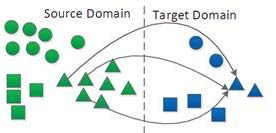## 统计特征变换

### 子空间对齐法 (Subspace Alignment, SA)

• 直接寻求一个线性变换,把source变换到target空间中
• 优化目标: $$F(M) = ||X_SM-X_T||^2_F$$ $$M^* = argmin_M(F(M))$$
• 直接获得线性变换的闭式解: $$F(M) = ||X_S’X_SM-X_S’X_T||^2_F = ||M-X_S’X_T||^2_F$$

### 关联对齐法 (CORrelation Alignment, CORAL)

• 最小化源域和目标域的二阶统计特征，其优化目标为：$\min_{A} = ||C_S - C_T||^2_F = \min_{A}||A^TC_sA-C_T||^2_F$
• 形式简单，求解高效

### 流形学习

#### 采样测地线留方法（Sample Geodesic Flow）

• 把领域自适应的问题看成一个增量式“行走”问题
• 从源域走到目标域就完成了一个自适应过程
• 在流形空间中采样有限个点,构建一个测地线流

#### 测地线流式核方法 (Geodesic Flow Kernel, GFK)

• 继承了SGF方法,采样无穷个点
• 转化成Grassmann流形中的核学习,构建了GFK
• 优化目标:$(z_i^\infty,z_j^\infty) = \int_0^1 (\Phi(t)^Tx_i)^T (\Phi(t)^Tx_j)^T dt = x_i^TGx_j$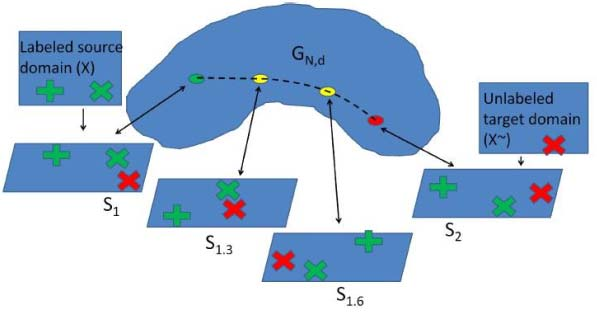#### 域不变映射 (Domain-Invariant Projection, DIP) [Baktashmotlagh,CVPR-13]

• 直接度量分布距离是不好的:原始空间特征扭曲
• 仅作流形子空间学习:无法刻画分布距离
• 解决方案:流形映射+分布度量

#### 统计流形法 (Statistical Manifold) [Baktashmotlagh, CVPR-14]

• 在统计流形(黎曼流形)上进行分布度量
• 用Fisher-Rao distance (Hellinger distance)进行度量

$$\min_\alpha = \dfrac{1}{\sum_{i=1}^{n_s}\alpha_i} \sum_{i=1}^{n_s} \alpha_i (\sqrt{T(x_i^s)}- \sqrt{1-T(x_i^s)} )^2 + \dfrac{1}{n_t} \sum_{i=1}^{n_s} (\sqrt{T(x_i^s)}- \sqrt{1-T(x_i^s)})^2$$

#### 总结

• 主要包括统计特征对齐和流形学习方法两大类
• 和分布适配结合效果更好
• 趋势:与神经网络结合

# 最新成果

• 与深度学习结合
• Deep Adaptation Networks (DAN) [Long, ICML-15] 深度网络+MMD距离最小化
• Joint Adaptation Networks (JAN) [Long, ICML-17] 深度网络+联合分布距离最小化
• Simultaneous feature and task transfer [Tzeng, ICCV-15] 特征和任务同时进行迁移
• Deep Hashing Network (DHN) [CVPR-17] 在深度网络中同时学习域适应和深度Hash特征
• Label Efficient Learning of Transferable Representations across Domains and Tasks [Luo, NIPS-17] 在深度网络中进行任务迁移
• 与对抗学习进行结合
• Domain-adversarial neural network 深度网络中加入对抗 [Ganin, JMLR-16]